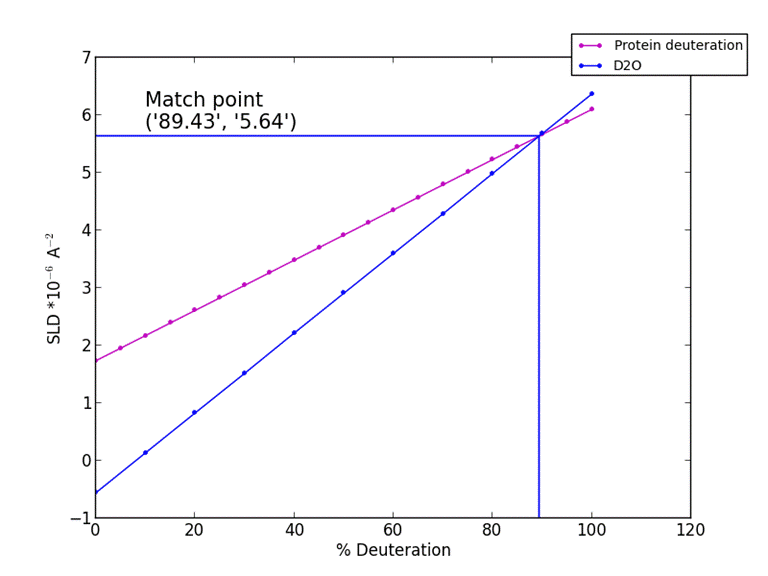Biomolecular Scattering Length Density Calculator

Introduction

The biomolecular neutron scattering length denisty calculator calculates a number of properties of a protein including the molecular weight (in kilodaltons), scattering length (in 10-4 Å) and scattering length density (in 10-6-2) of a protein from it's primary structure (in single letter format) based on user defined sample variables such as solution D2O content (in % of total solution volume), % deuteration (0-100%) and % labile hydrogen exchange (0-100%). .

The purpose of this application is to automate calculations of protein neutron scattering properties and therefore remove the need for laborious "manual" calculations.

Theory

A proteins scattering length (b) can be calculated as the sum of the scattering length of each individual amino acid within a polypeptide, which in turn, can be calculated from the sum of the scattering length of each element within a given residue. The scattering length of each amino acid will vary with both the solution D2O concentration (due to the exchange of labile hydrogen's) and % deuteration of non-exchangeable hydrogen's when a protein/peptide is deuterated. The protein neutron scattering properties calculator allows you to state your solution and sample conditions (which affect both the proteins scattering length and molecular weight) which will determine the scattering length of the protein. The proteins volume can also be determined as the sum of the volumes of the individual amino acids (in the case of this program, volumes obtained from a consensus of a number of techniques (Perkins, S, Eur. J. Biochem, 1986, 157, 169-180.)). The amino acid scattering lengths for hydrogenated amino acids in H2O and D2O, and deuterated in D2O were obtained from "The study of biological molecules by neutron scattering" by B Jacrot (Rep. Prog. Phys. 1976 39 911-953). The nucleotide scattering length and volumes were taken from the book "Neutron scattering in biology: Techniques and Applications" (pg 15, ISSN: 1618-7210). The deuterated values in H2O were manual calculated from the sum of the corresponding atoms. The table of the published values are given below.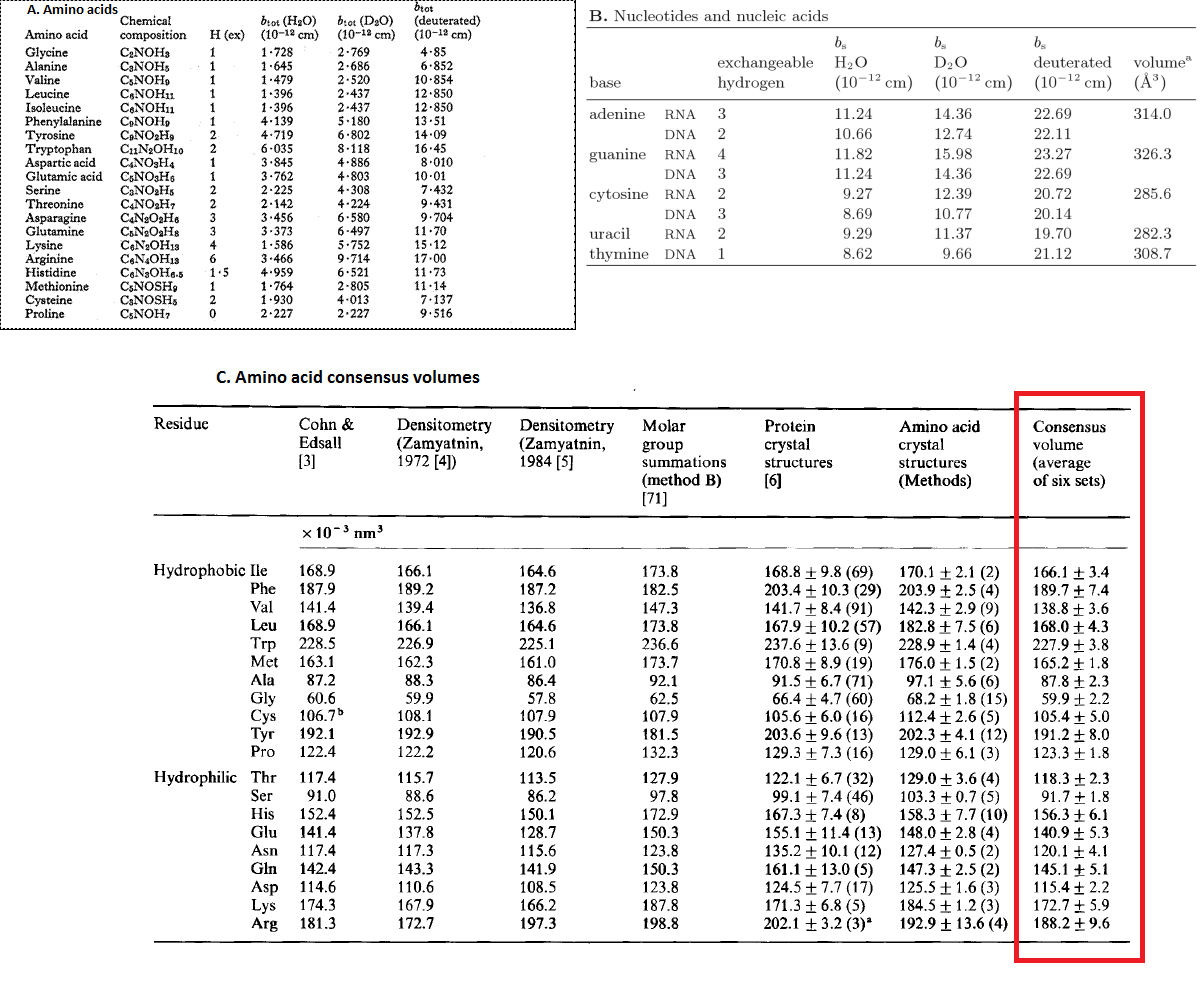The scattering length density of a molecule is merely the scattering length (Σb) divided by its volume: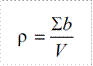Where ρ is the scattering length density, Σb is the proteins scattering length and v is its volume.

Starting up and user interface

Below is a screen shot of websites input screen, with input boxes and buttons labelled from 1-4: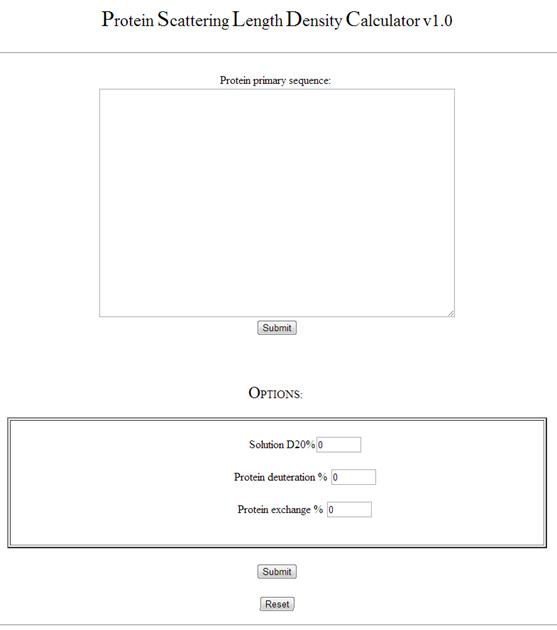The text boxes and buttons are grouped into 4 different types

Input variables (1-4)

1. Protein primary structure input. Copy your protein primary structure from source (if you want to find the primary structure try the European bioinformatics website (http://www.ebi.ac.uk/)). Once you have copied the structure click on the "Protein primary structure box" and press Ctrl-V to input your sequence.

2. Solution D2O % input. Input for the % volume of D2O in your aqueous solution. For example a pure D2O solution would be 100% and an air contrast matched water (ACMW) solution would be 8%.

3. Protein Deuteration % input. If you have deuterated your protein sample and you know the % deuteration from either mass spectrometry or contrast matching data you can input this. For regular hydrogenated protein samples input 0 into this text box.

Exchange 4. Hydrogen / Deuterium exchange %. For a hydrogenated protein in a 100% D2O solution there is considerable exchange of side chain and amide backbone hydrogens with the solution. This changes the molecular weight of the protein in a given solution. The exchange term is dependant upon the exposure of the proteins hydrogens to the solution. This is dependant on the proteins conformation. The value can estimated to be ~ 90% hydrogens exposed to solution, but can vary depending on the protein of interest. It can also be determined experimentally from NMR, mass spectrometry or infra-red spectroscopy. Note: The exchange term assumes the concentration of the solution is greatly in excess of the protein, which is usual in biological experiments.

Submit (4). Press to calculate the protein neutron scattering properties from the data input.

Output page (6-8)

The output page details a number of chemical components of the sequence entered, as shown with the example sequence below: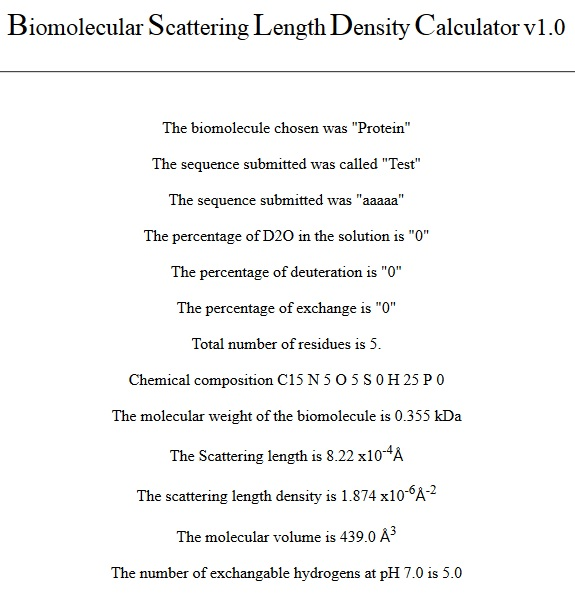5. Protein sequence name and sequence submitted

6. Inputted values: percentage D2O, deuteration and percentage of protein exchange.

7. Molecular weight, total number of amino acids and chemical composition output. This outputs the molecular weight of the protein based on its sequence and the level of deuteration of the solvent and the protein.

8. Protein molecular volume. This outputs the molecular volume of the protein based on the sum of the volumes of its constituent amino acids from its primary sequence.

9. The total number of hydrogens and those that are solvent exchangeable

10. Scattering length and scattering length density output. This contains the total scattering length of the proteinb) based on its sequence and the level of deuteration of the solvent and the protein in angstroms (Å). This value is then divided by the molecular volume calculated and outputted as the scattering length density of the protein

11. Estimate of Zero angle (forward) intensity. This is a theoretical calculation of the intensity of scatter using the equation: I(0) = φV (ρp-ρw), where φ is the voulumr fraction, V is the volume of the particle, ρp is the  scattering length density of the particle and  ρw is the scattering length density in the solvent.

The output graphs

9. Output protein scattering length density vs. D2O % graph.

A graph of how the protein at your set deuteration level will change scattering length density against D2O solution %.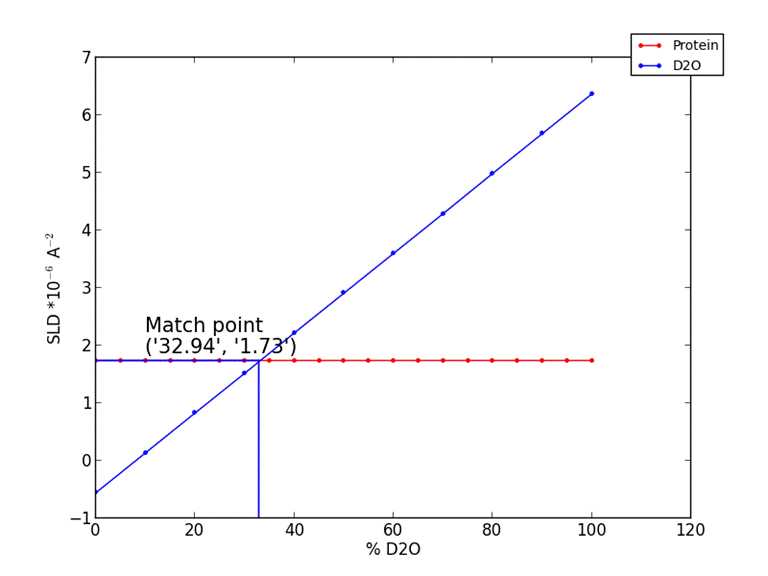10. Output protein scattering length density vs. % deuteration

A graph of how the protein at your set solution D2O % will change scattering length density against protein deuteration.